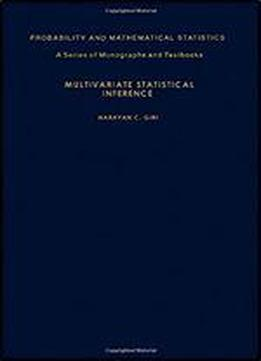# Multivariate Statistical Inference by Narayan C. Giri / 1977 / English / PDF

Multivariate Statistical Inference is a 10-chapter text that covers the theoretical and applied aspects of multivariate analysis, specifically the multivariate normal distribution using the invariance approach. Chapter I contains some special results regarding characteristic roots and vectors, and partitioned submatrices of real and complex matrices, as well as some special theorems on real and complex matrices useful in multivariate analysis. Chapter II deals with the theory of groups and related results that are useful for the development of invariant statistical test procedures, including the Jacobians of some specific transformations that are useful for deriving multivariate sampling distributions. Chapter III is devoted to basic notions of multivariate distributions and the principle of invariance in statistical testing of hypotheses. Chapters IV and V deal with the study of the real multivariate normal distribution through the probability density function and through a simple characterization and the maximum likelihood estimators of the parameters of the multivariate normal distribution and their optimum properties. Chapter VI tackles a systematic derivation of basic multivariate sampling distributions for the real case, while Chapter VII explores the tests and confidence regions of mean vectors of multivariate normal populations with known and unknown covariance matrices and their optimum properties. Chapter VIII is devoted to a systematic derivation of tests concerning covariance matrices and mean vectors of multivariate normal populations and to the study of their optimum properties. Chapters IX and X look into a treatment of discriminant analysis and the different covariance models and their analysis for the multivariate normal distribution. These chapters also deal with the principal components, factor models, canonical correlations, and time series.

views: 659